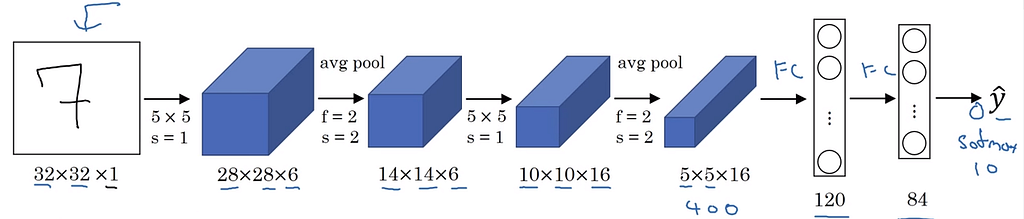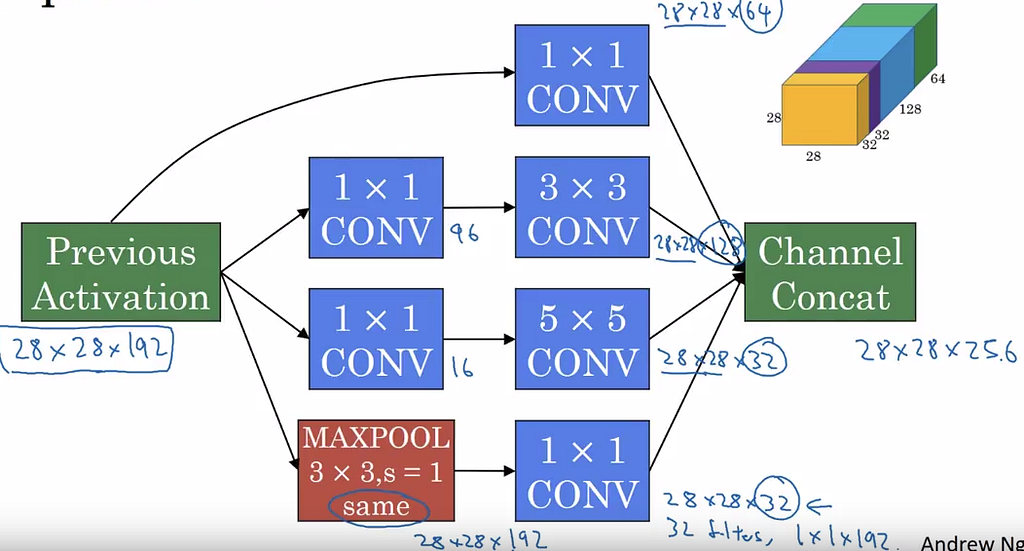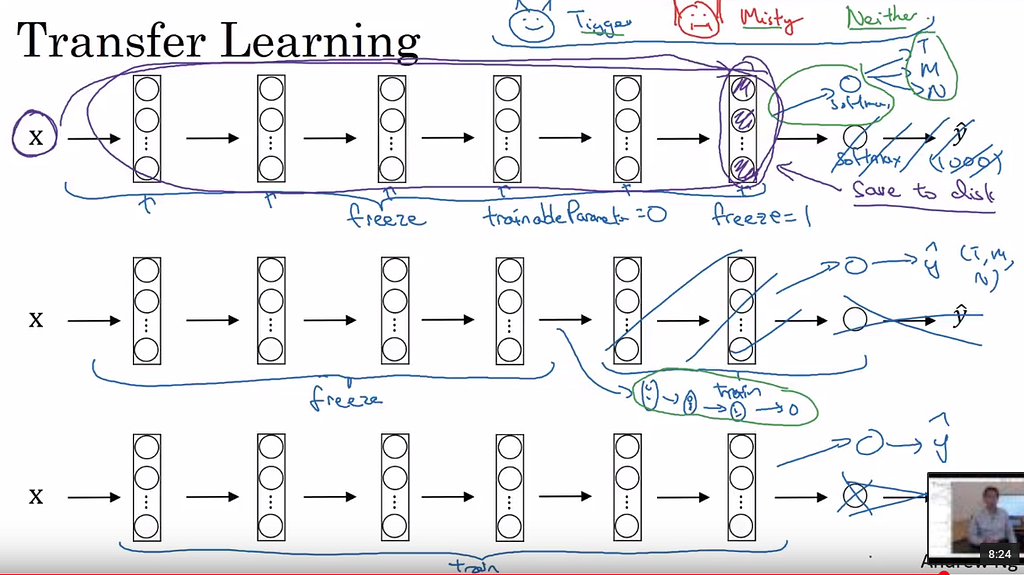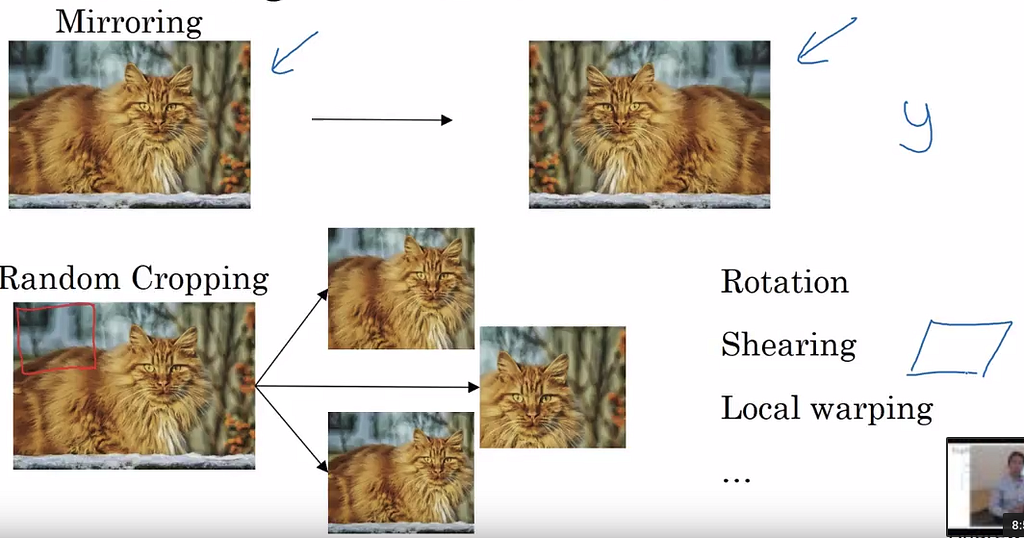# Deeplearning.ai: CNN week 2

#### Case studies of CNN

• How to put CNN components together ?
• Learn from other source code/ CNN architecture.
• LeNet-5, AlexNet, VGG, ResNet, Inception neural net.
• Ideas from one application/artchitecture can transfer to others domain.

#### Classic network

• Letnet-5: grayscale image of numbers (32 x 32) -> two times of filtering and pooling (4 layers)→ 2 layers of fully connected → soft max func → labels. Purpose to recognize the numbers.
• AlexNet: almost the same as LeNet, extend more layers and parameters in the scale of milions.
• VGG-16: all Conv and max-poll in the network are the same settings. But the network is very large, larger than the AlexNet. Interestingly, while the max pooling decrease the size of the data “tensor”, the number of filter is increasing according to the scale of 2.

#### Resnet

• Vanishing, exploding gradient infomation ? How to train very deep neural network ?
• A residual block. Typically, the input will be manipulated under two linear transformation with Relu activation. A residual network is copy the input directly to the second transformation output, and feed the sum into the final Relu.
• Residual Network: add many residual block together. The plain very deep network gradually loss its robust after a certain deepth, increasing the error. Nevertheless, the ResNet is not faced this problem.

#### Why ResNet work ?

• Let think about the learning scenario: If W[l+1] = 0 and b[l+1] = 0, then the ouput a[l+2] = a[l] and thus “g” is the identity function. Recall that the Relu filters out negative values of a[l].
• Then in the worst case of learning nothing or zero weights, the network still can learn the identiy function. That we know at least one solution for the network.
• In the “Plain” network, if W[l+1] = 0 and b[l+1] = 0, then a[l+2] = 0. Of course, when the weight vanishing, nothings meaningful returned. We do not know the “worst” solution.
• Then the “residual” means learning somethings residual/added transformation of the actual input but useful. The “Plain” network simply learns the serial transformation of input via layers.

#### Network in Network

• 1 x 1 convolution. Technically, 1×1 conv operator in a matrices is the amplification of input data. BUT, it is worth when the input data is tensors, for example: the RBG image.
• A filter now has the size of 1x1x “depth”, according to the 3rd dimension of data tensor. Taking the conv operator (element-wise multiplication over 3rd dimension) → sum of → ReLu. It is the same working way in a 1 output node network. Then it returns a number when applying 1×1 convolution in one “yellow” slide of the data tensor. Despite of the “depth” of the tensor, the output is a matrices: the Relu of linear transformation by the 1x1x “depth” convolution over tensor slides.
• When many filters are applied, the output “depth” is the number of filters. It is the responses of data tensor over each convolution configuration. Or the responses of different linear transformation. It makes me remember about machine learning with random projection, used to 5 or 6 years ago is a hot topic. Or Extreme Machine Learning :).
• The different with >1 size convo is the 1×1 keeps the origin size of 2 dimension. Then it decreases the number of channels, saving computation time. Simple but strength :).

#### Inception network

• Tough in choosing pool or convo or hyper parameters ?
• Let do all of them on the input data and stack the results together.
• Computational cost is a problem. For 32 filters of size 5×5 on the example input of 28x28x192 → computer needs to do 120milions calculation.
• BUT 1×1 convolution is our friend. Let reduces the number of input layers or “depth” first and do the “inception” later. It is the “bottle-net” network.
• Applying 16 filters of the size 1x1x192 to reduce the input tensor to 28x28x16 and continue with the convolution of 5×5….
• Reducing from 120milions calucation to 2.4m + 10.0m = 12.4m. Around 10 times reduce.
• Recap: do them all + concatenate
• An Inception moduleA typical module/ building block of the inception module. Source: deeplearning.ai C4W2L07
• I noticed that when the size of convolution filter increases, there are less number of filters. Maybe more “actions” to digging “small” details.
• Can add some softmax node in the middle to do the predicting task.

#### Using Opensource implementation

• Hard to replicate only with the publication of deep learning paper.
• It is easier to look online to find the author implementation.
• Google of Network “keyword + github”

#### Transfer learning

• Many open dataset, sources code, pretrained model → can use the initial weight → train in new domain.
• For example:3 ways of doing the transfer learning. . Source: deeplearning.ai C4W2L09
• IF you want to train a classifier to classifer images which contain “cat” names Tigger, Misty, and either.
• Which is the fastest way to train it with less available data about them?
• Let use the trained weights on big datasets, e.g: ImageNet, as the input weight and network structure for our purpose. There are 3 ways to utilize it:
• 1. Use the whole trained weights, “frozen” it (no retraining), use the output of final network layer, add a fully connected softmax layer and learn the classification weights of it. This way we are using the distance from a datapoint to other “well-known” class, as the feature to learn.
• 2. Almost the same as the first way, execept retrain some final layers, use the loaded weights as the initial points.
• 3. Retraining whole network with initial loaded weights. Of course, this can happen as we expand or modify the classes cluster “structure” rather than find the structure of them from beginning.
• Recap: save time and resources, faster develop.

#### Data Augmentation

• Training a computer vision task quite tough in term of data availability. How to create more data ?
• In common, Mirroring, Random croppingCropping and mirroring to create new data . Source: deeplearning.ai C4W2L10
• Color shifting
• Implement the data distortion during training: